Courses

# Thermodynamic Potential NAT Level – 1

## 10 Questions MCQ Test Topic wise Tests for IIT JAM Physics | Thermodynamic Potential NAT Level – 1

Description
This mock test of Thermodynamic Potential NAT Level – 1 for IIT JAM helps you for every IIT JAM entrance exam. This contains 10 Multiple Choice Questions for IIT JAM Thermodynamic Potential NAT Level – 1 (mcq) to study with solutions a complete question bank. The solved questions answers in this Thermodynamic Potential NAT Level – 1 quiz give you a good mix of easy questions and tough questions. IIT JAM students definitely take this Thermodynamic Potential NAT Level – 1 exercise for a better result in the exam. You can find other Thermodynamic Potential NAT Level – 1 extra questions, long questions & short questions for IIT JAM on EduRev as well by searching above.
*Answer can only contain numeric values
QUESTION: 1

### A system of N localized, non-interacting spin 1/2 ions of magnetic moment in each is kept in an external  magnetic field H. If the system is in equilibrium at temperature T, the Helmholtz free energy of the system is given as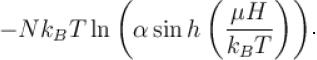Calculate the value of α?

Solution:

Partition function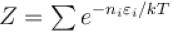Vibrational partition function in this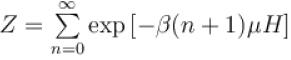where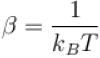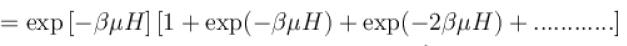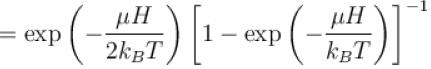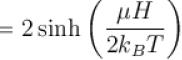For the whole system  Z(v) = [Z(v)]N
Helmholtz free energy F = –NkB TlnZ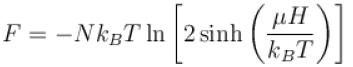⇒ α = 2

*Answer can only contain numeric values
QUESTION: 2

### The free energy of a photon gas enclosed in a volume V is given by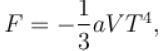where a is the constant and T is the temperature of the gas. The chemical potential of the photon gas is?

Solution: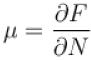and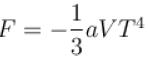No term depends on N
hence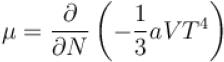µ = 0

*Answer can only contain numeric values
QUESTION: 3

### Calculate the standard Gibb’s free energy change for the formation of methane from carbon and hydrogen at 298 K in the units of kJ/mol. Given that ΔH is –74.9 kJ/mol and ΔS = -80.75J{\rm{/K - mol}}.\)

Solution:

Given  ΔH = –74.9 kJ/mol
ΔS = –80.75 J/K-mol
Now use the equation
ΔS = ΔH – TΔS
⇒ ΔS = –74.9 kJ/mol +298 K (80.75/K-mol) × (1 kJ/1000J)
⇒ ΔS = – 50.9 kJ/mol

*Answer can only contain numeric values
QUESTION: 4

Calculate the depression of melting point of ice (in K) produced by 1 atm increase of pressure, given that Lice = 80 cal gm-1 and specific volume of ice and water at 0°C are 1.091 cm3 and 1 cm3 respectively

Solution:

1 atm = 105 N/m2
= 106 dynes/cm2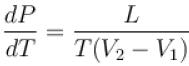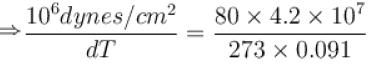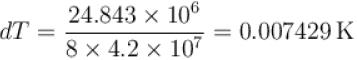*Answer can only contain numeric values
QUESTION: 5

To change the melting point of ice by 1K, what should be the change in the pressure, given that L = 80 cal gm-1 and specific volume of ice and water at 0°C are 1 cm3 and 1.091 cm3 respectively. Give your answer in atm.

Solution: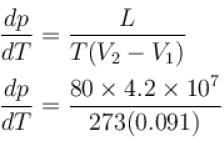dT = 1K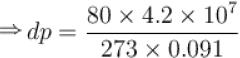dp = 1.3525 × 108 dynes/cm2
⇒dp = 135.25 atm.

*Answer can only contain numeric values
QUESTION: 6

Calculate the value of ΔH for an isothermal process?

Solution:

ΔH = Cp ΔT
ΔT = 0
⇒ ΔH = 0

*Answer can only contain numeric values
QUESTION: 7

If Z be the partition function and N represent number of particles the chemical potential is given as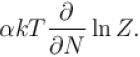Find the value of α.

Solution:

dF = dU – pdV + µdN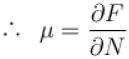Since F = –kT ln Z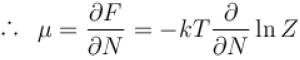⇒ α = –1

*Answer can only contain numeric values
QUESTION: 8

A solid melts into liquid. The relation between the pressure p and the temperature T of the phase transition is P = –2T + P0 , the entropy charge associated with the phase transition is 1 Joule mol-1 K-1 . The Claussius-Clapeyron equation for the latent heat latent heat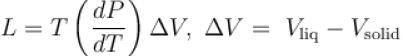then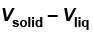is equal to?

Solution:

p = –2T + p0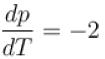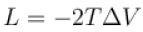ΔS = 1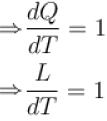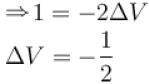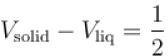*Answer can only contain numeric values
QUESTION: 9

It the partition function of a harmonic oscillator with frequency  at temperature T in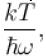then the free energy of 8 such independent oscillators in terms of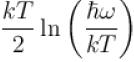is

Solution:

The Helmholtz free energy N independent harmonic oscillator in terms of partition function Z is
F = –NkT ln Z
here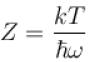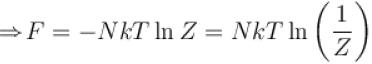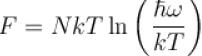N = 8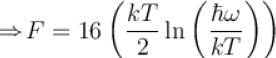*Answer can only contain numeric values
QUESTION: 10

A system of N non-interacting and distinguishable particles of spin1 is in thermodynamic equilibrium. The entropy of the system turns out to be of the form NkBln x. Find the value of x?

Solution:

Number of microstates  Ω = 3N
Entropy is given by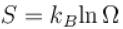∴ S = NKBln 3
⇒ x = 3